# RD Sharma Solutions for Class 11 Chapter 33 - Probability Exercise 33.4

Until now we have been computing the probability of occurrence or non-occurrence of an event by using favourable and total number of elementary events. Theorems, which express the probability of an event in terms of the probabilities of those events whose union is the given event, are known as addition theorems on probability. The solutions are explained by the subject experts in simple language to help students improve their analytical thinking and problem solving abilities. RD Sharma Class 11 Maths Solutions pdf are available for free download to boost their exam preparation and score well in their board exams.

## Download the pdf of RD Sharma Solutions for Class 11 Maths Exercise 33.4 Chapter 33 – Probability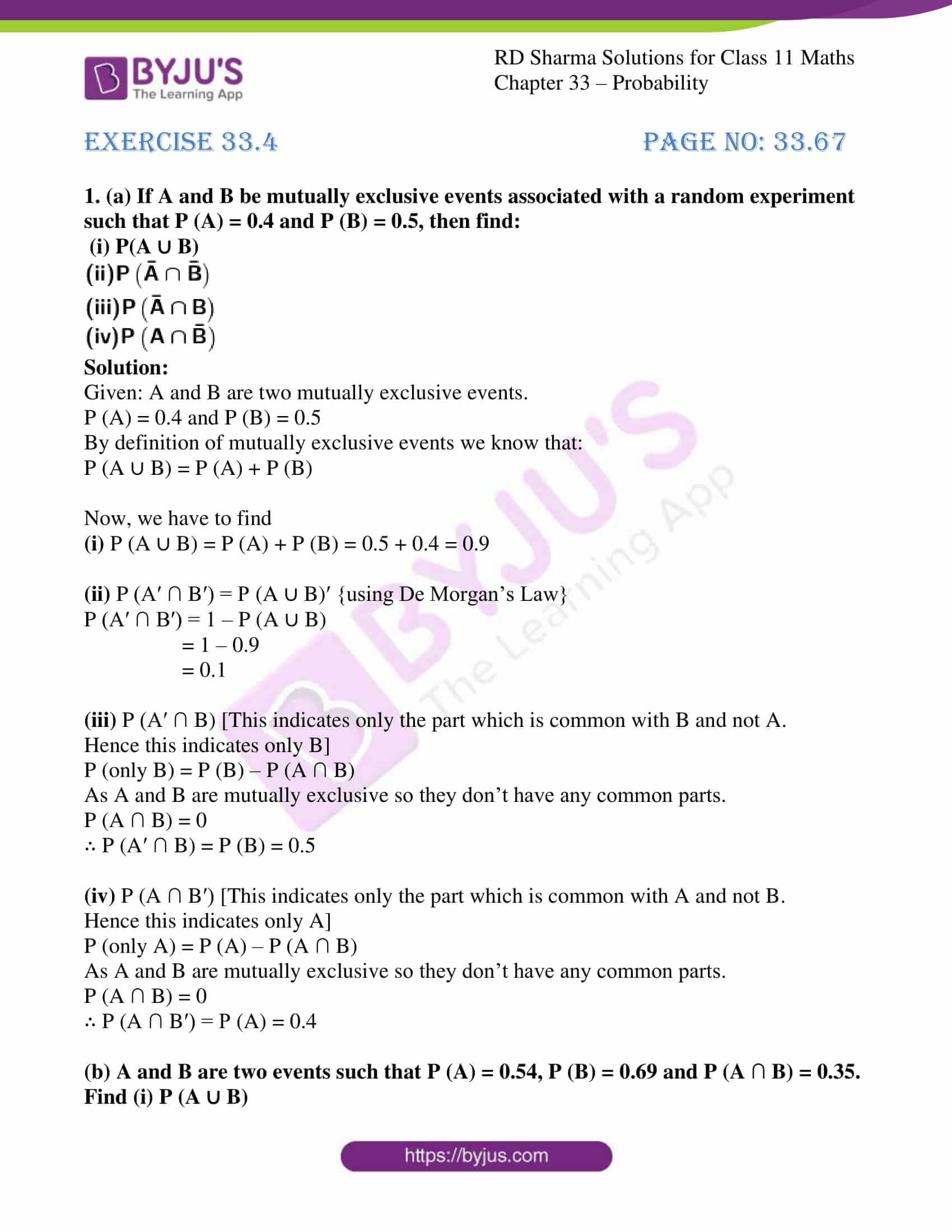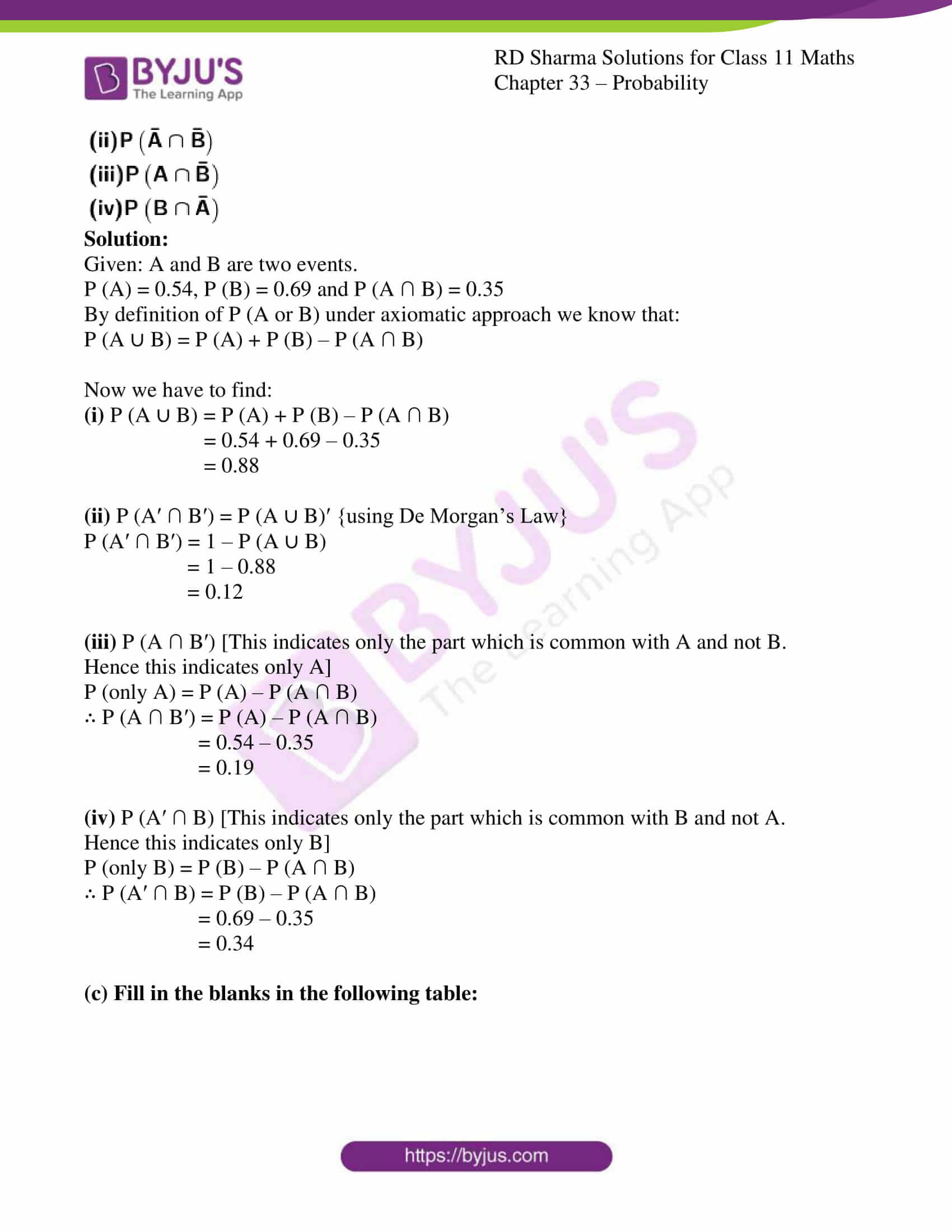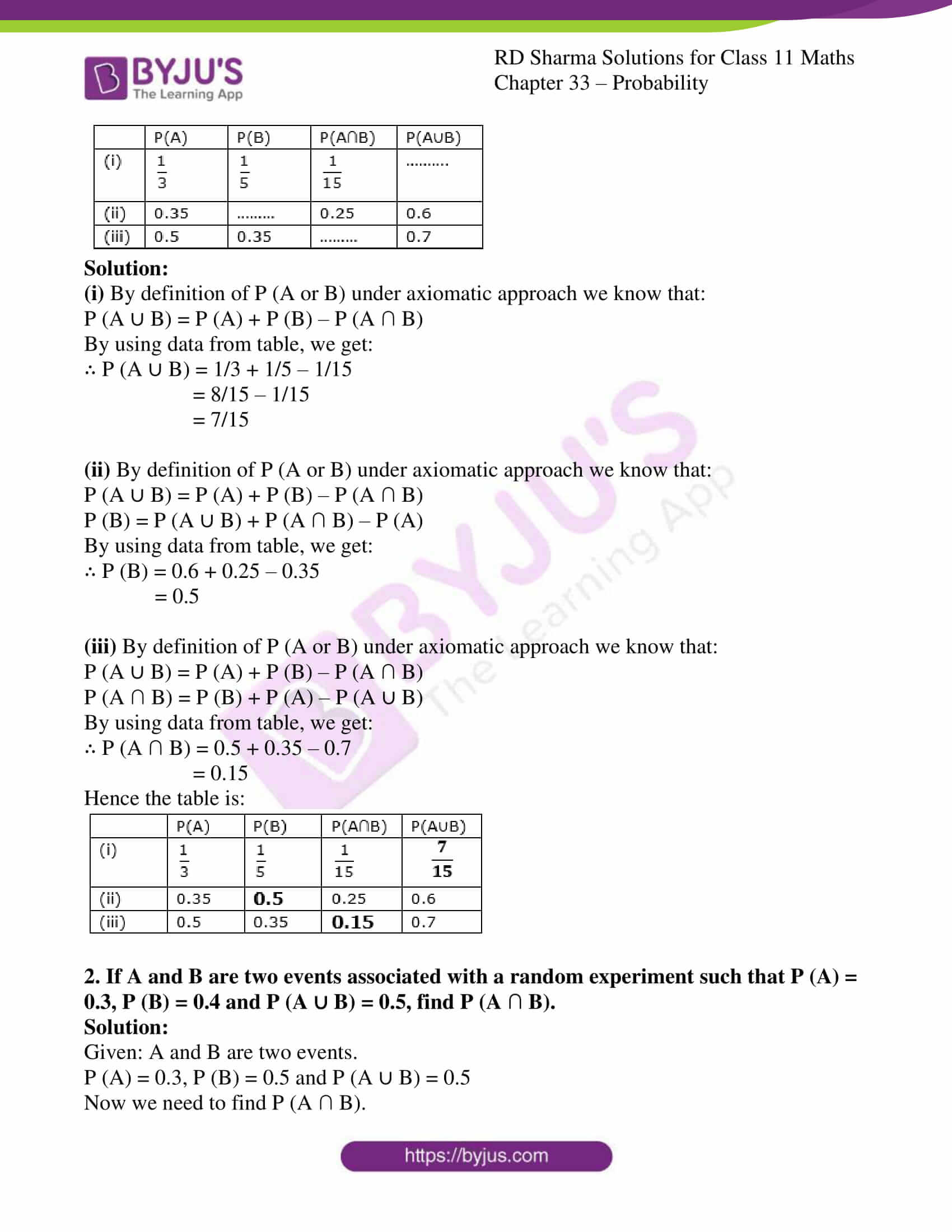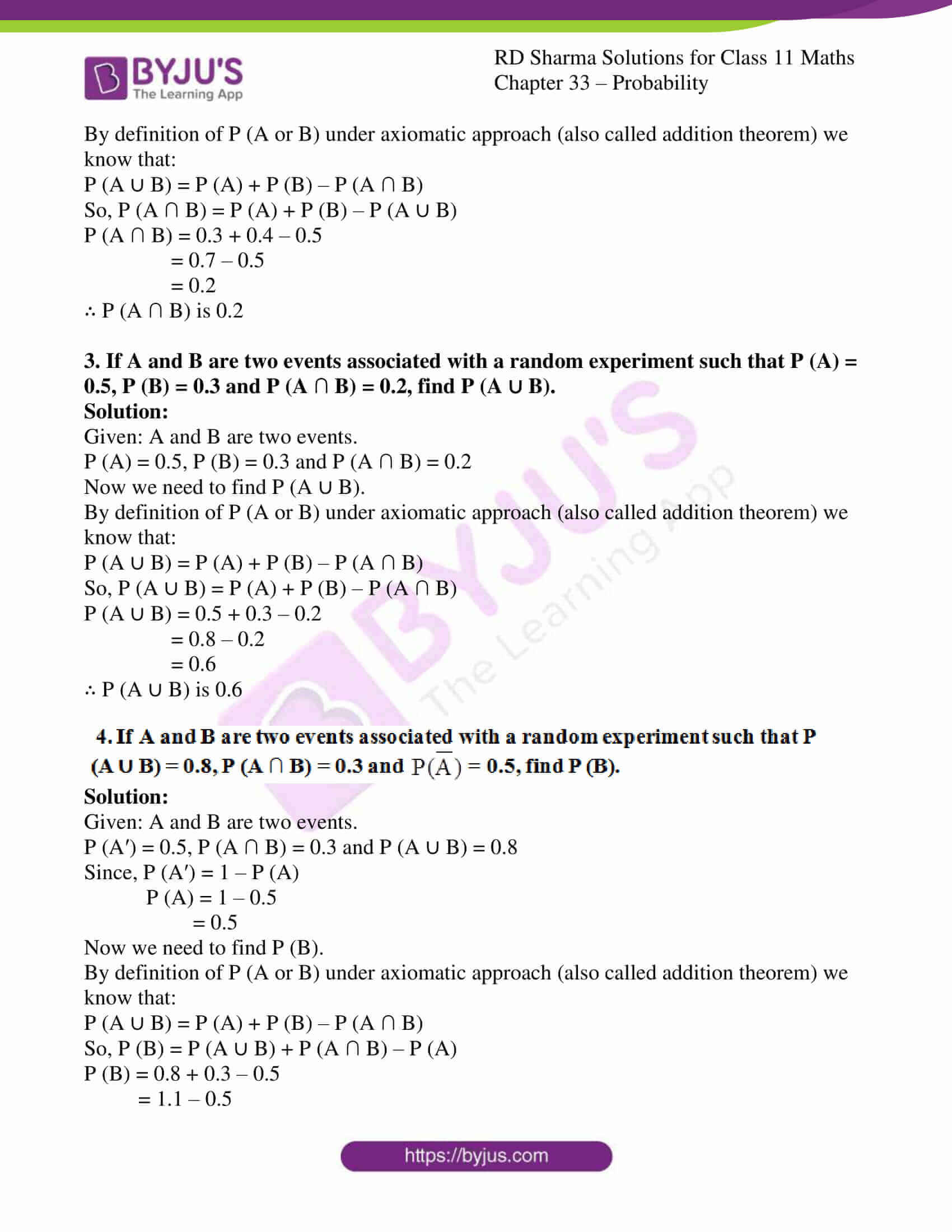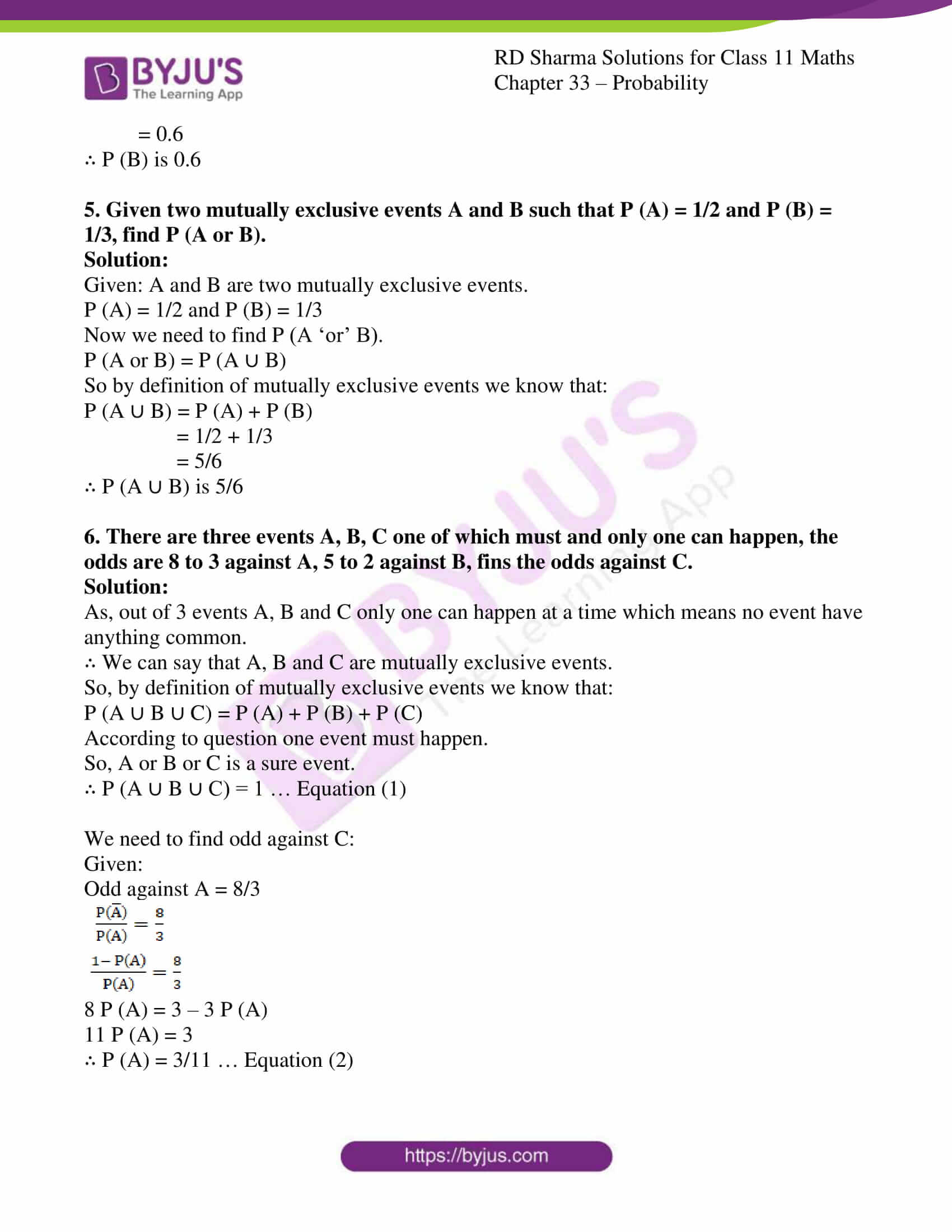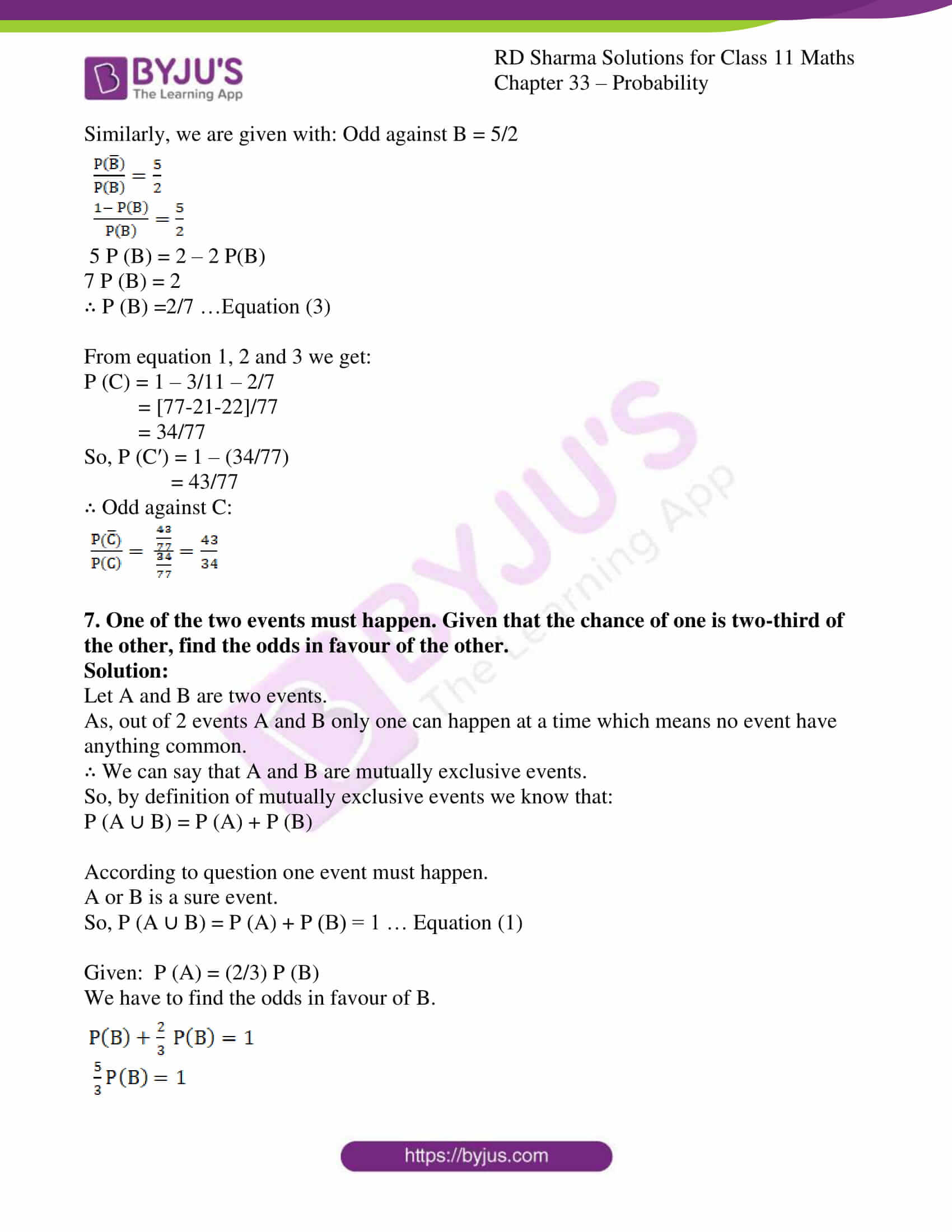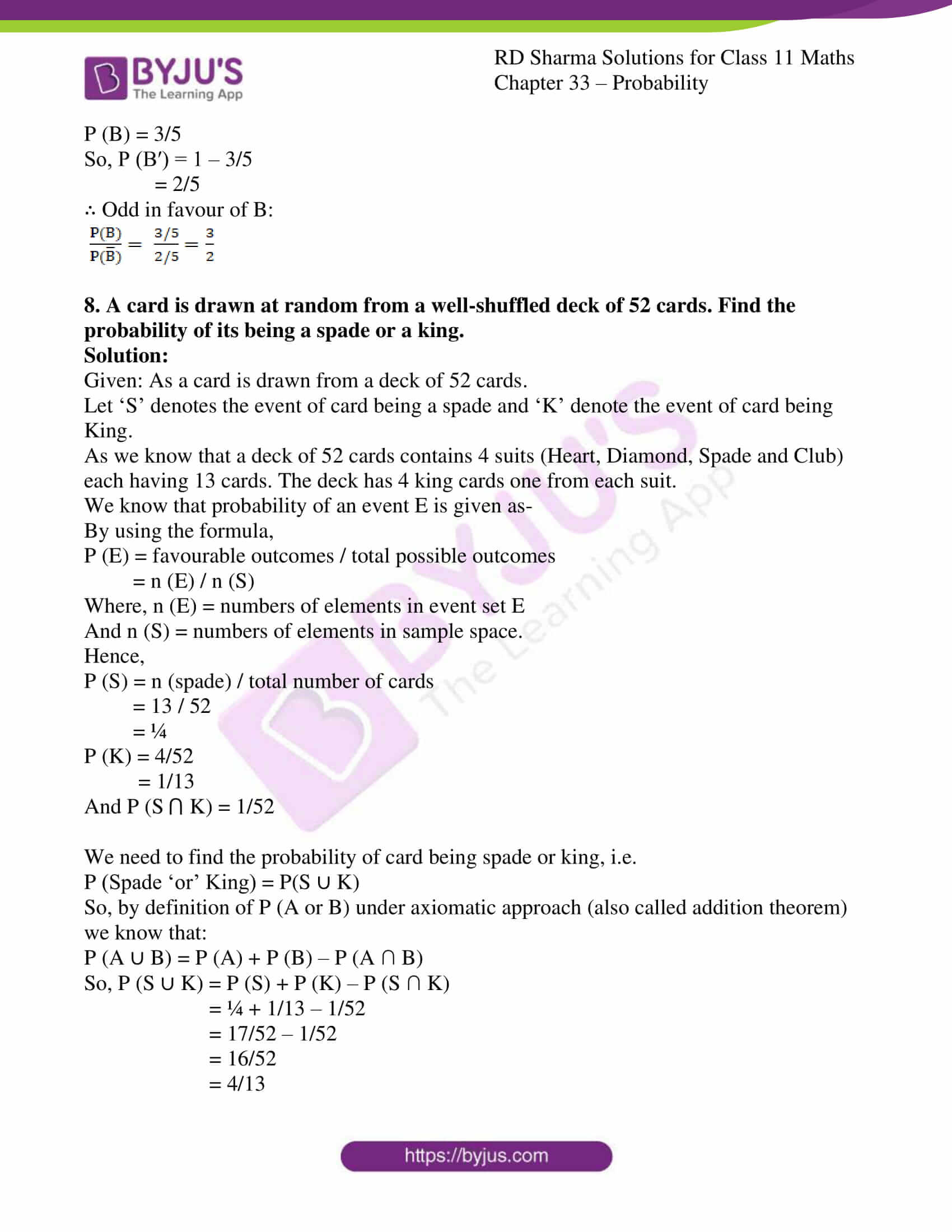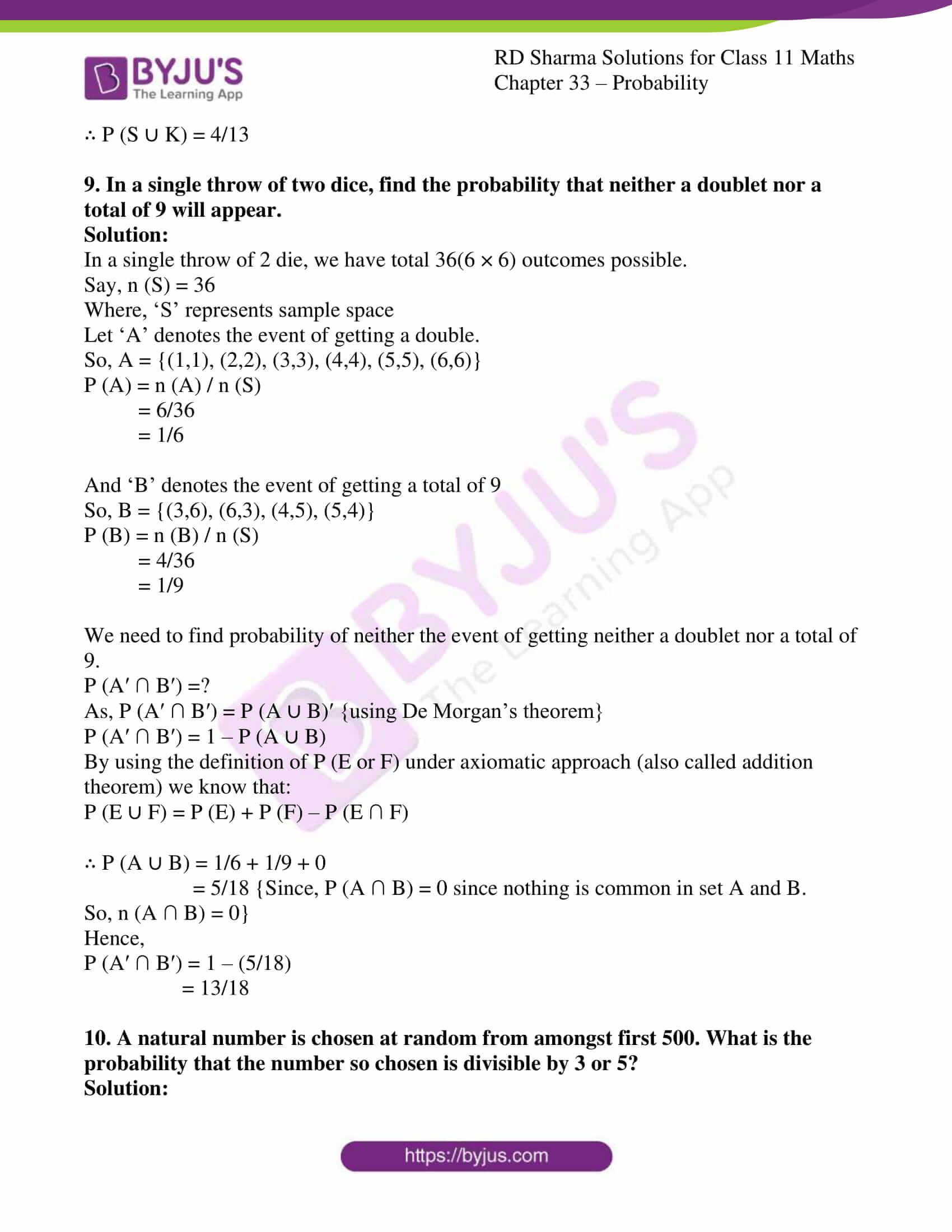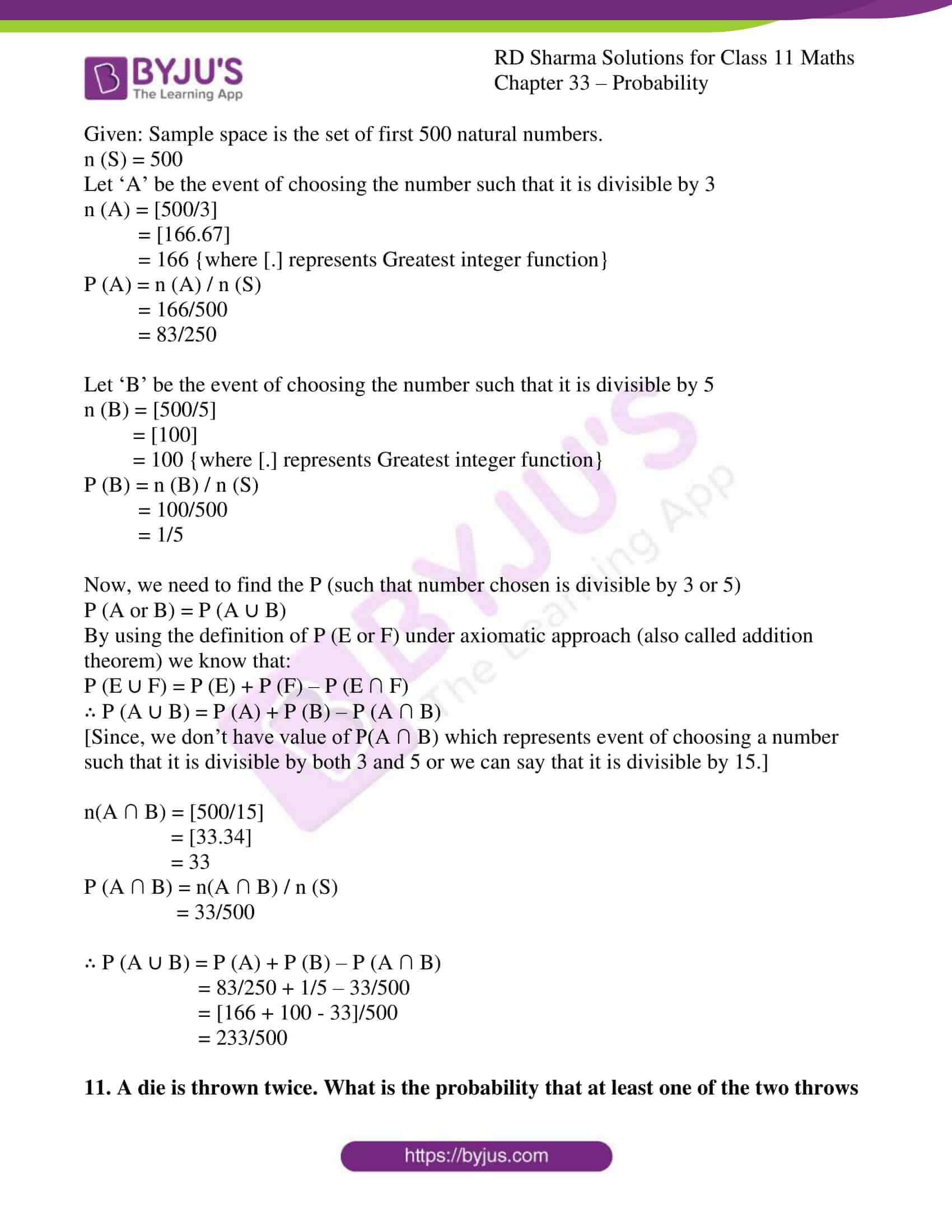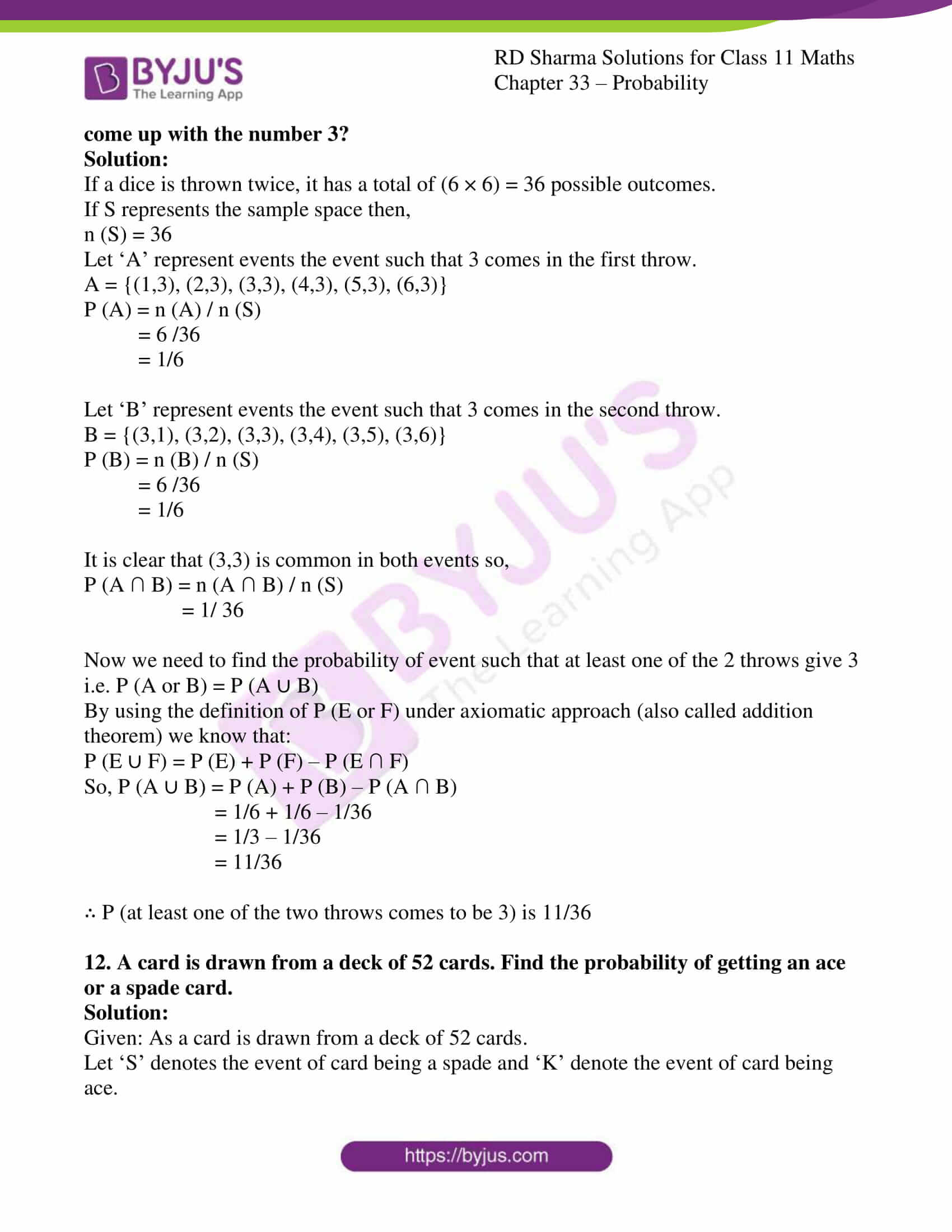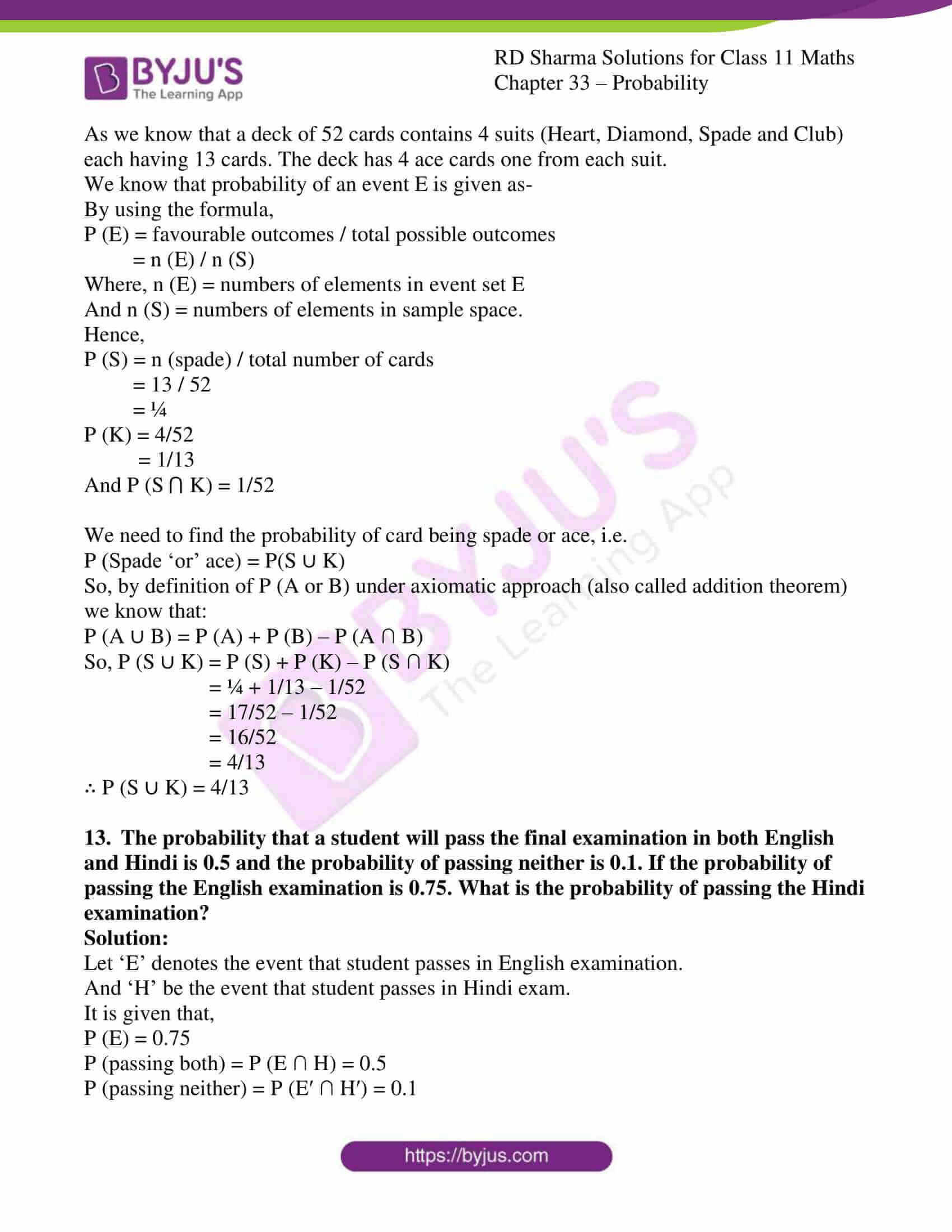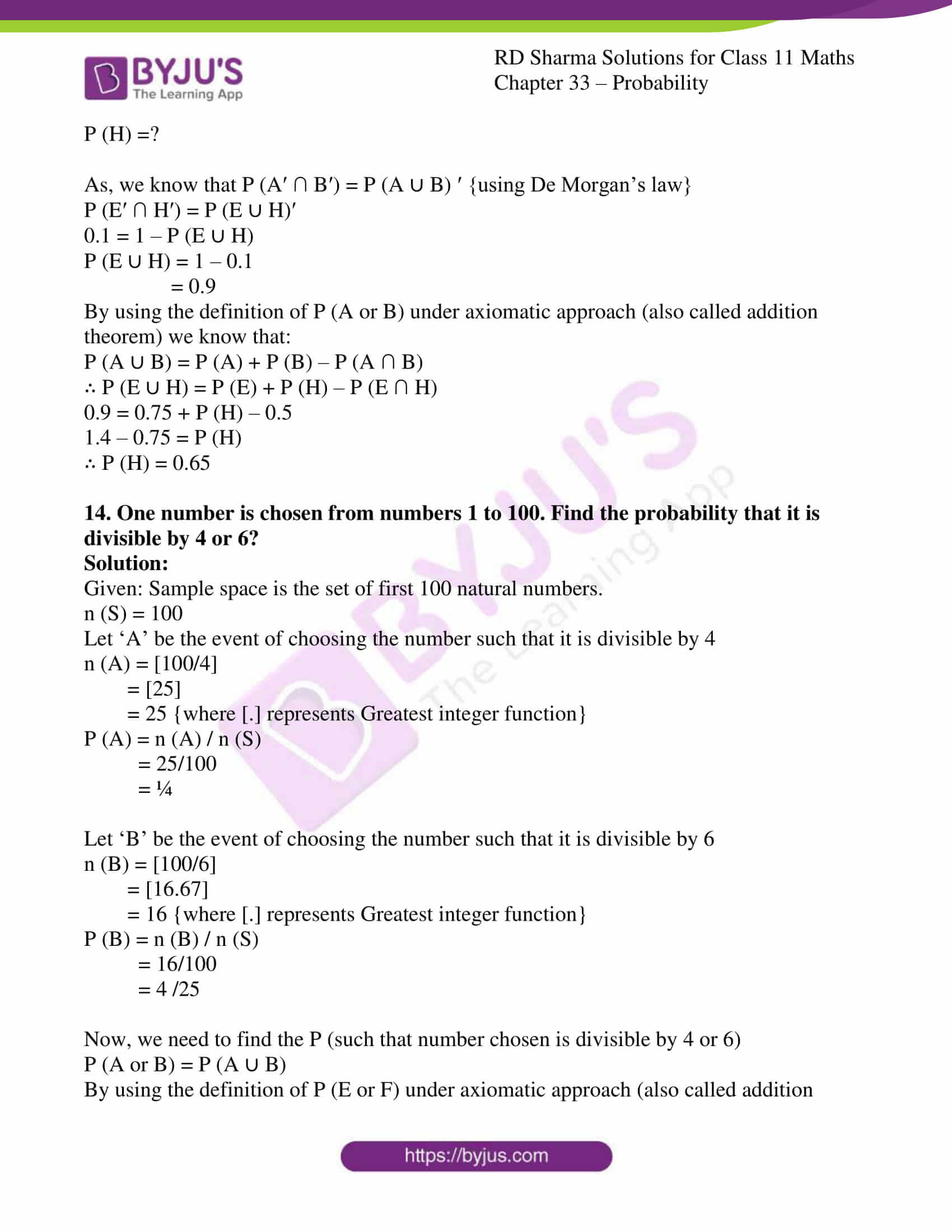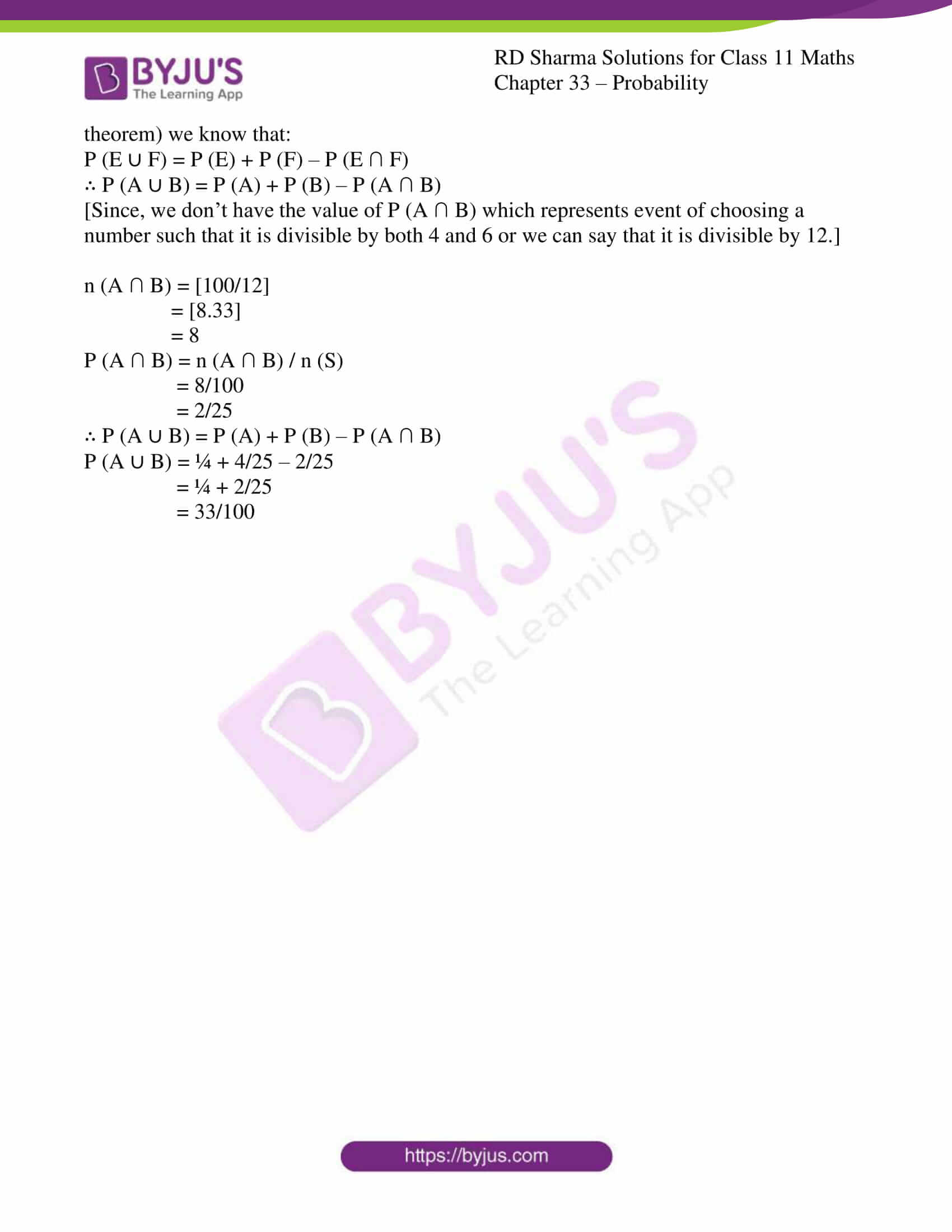### Access answers to RD Sharma Solutions for Class 11 Maths Exercise 33.4 Chapter 33 – Probability

#### EXERCISE 33.4 PAGE NO: 33.67

1. (a) If A and B be mutually exclusive events associated with a random experiment such that P (A) = 0.4 and P (B) = 0.5, then find:
(i) P(A ∪ B)Solution:

Given: A and B are two mutually exclusive events.

P (A) = 0.4 and P (B) = 0.5

By definition of mutually exclusive events we know that:

P (A ∪ B) = P (A) + P (B)

Now, we have to find

(i) P (A ∪ B) = P (A) + P (B) = 0.5 + 0.4 = 0.9

(ii) P (A′ ∩ B′) = P (A ∪ B)′ {using De Morgan’s Law}

P (A′ ∩ B′) = 1 – P (A ∪ B)

= 1 – 0.9

= 0.1

(iii) P (A′ ∩ B) [This indicates only the part which is common with B and not A.

Hence this indicates only B]
P (only B) = P (B) – P (A ∩ B)

As A and B are mutually exclusive so they don’t have any common parts.

P (A ∩ B) = 0

∴ P (A′ ∩ B) = P (B) = 0.5

(iv) P (A ∩ B′) [This indicates only the part which is common with A and not B.

Hence this indicates only A]

P (only A) = P (A) – P (A ∩ B)

As A and B are mutually exclusive so they don’t have any common parts.

P (A ∩ B) = 0

∴ P (A ∩ B′) = P (A) = 0.4

(b) A and B are two events such that P (A) = 0.54, P (B) = 0.69 and P (A ∩ B) = 0.35. Find (i) P (A ∪ B)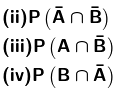Solution:

Given: A and B are two events.

P (A) = 0.54, P (B) = 0.69 and P (A ∩ B) = 0.35

By definition of P (A or B) under axiomatic approach we know that:

P (A ∪ B) = P (A) + P (B) – P (A ∩ B)

Now we have to find:

(i) P (A ∪ B) = P (A) + P (B) – P (A ∩ B)

= 0.54 + 0.69 – 0.35

= 0.88

(ii) P (A′ ∩ B′) = P (A ∪ B)′ {using De Morgan’s Law}

P (A′ ∩ B′) = 1 – P (A ∪ B)

= 1 – 0.88

= 0.12

(iii) P (A ∩ B′) [This indicates only the part which is common with A and not B.

Hence this indicates only A]

P (only A) = P (A) – P (A ∩ B)

∴ P (A ∩ B′) = P (A) – P (A ∩ B)

= 0.54 – 0.35

= 0.19

(iv) P (A′ ∩ B) [This indicates only the part which is common with B and not A.

Hence this indicates only B]

P (only B) = P (B) – P (A ∩ B)

∴ P (A′ ∩ B) = P (B) – P (A ∩ B)

= 0.69 – 0.35

= 0.34

(c) Fill in the blanks in the following table: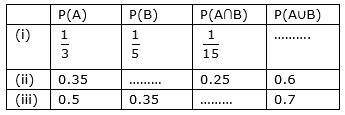Solution:

(i) By definition of P (A or B) under axiomatic approach we know that:

P (A ∪ B) = P (A) + P (B) – P (A ∩ B)

By using data from table, we get:

∴ P (A ∪ B) = 1/3 + 1/5 – 1/15

= 8/15 – 1/15

= 7/15

(ii) By definition of P (A or B) under axiomatic approach we know that:

P (A ∪ B) = P (A) + P (B) – P (A ∩ B)

P (B) = P (A ∪ B) + P (A ∩ B) – P (A)

By using data from table, we get:

∴ P (B) = 0.6 + 0.25 – 0.35

= 0.5

(iii) By definition of P (A or B) under axiomatic approach we know that:

P (A ∪ B) = P (A) + P (B) – P (A ∩ B)

P (A ∩ B) = P (B) + P (A) – P (A ∪ B)

By using data from table, we get:

∴ P (A ∩ B) = 0.5 + 0.35 – 0.7

= 0.15

Hence the table is: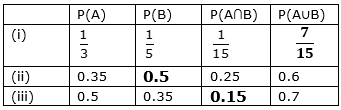2. If A and B are two events associated with a random experiment such that P (A) = 0.3, P (B) = 0.4 and P (A ∪ B) = 0.5, find P (A ∩ B).

Solution:

Given: A and B are two events.

P (A) = 0.3, P (B) = 0.5 and P (A ∪ B) = 0.5

Now we need to find P (A ∩ B).

By definition of P (A or B) under axiomatic approach (also called addition theorem) we know that:

P (A ∪ B) = P (A) + P (B) – P (A ∩ B)

So, P (A ∩ B) = P (A) + P (B) – P (A ∪ B)

P (A ∩ B) = 0.3 + 0.4 – 0.5

= 0.7 – 0.5

= 0.2

∴ P (A ∩ B) is 0.2

3. If A and B are two events associated with a random experiment such that P (A) = 0.5, P (B) = 0.3 and P (A ∩ B) = 0.2, find P (A ∪ B).

Solution:

Given: A and B are two events.

P (A) = 0.5, P (B) = 0.3 and P (A ∩ B) = 0.2

Now we need to find P (A ∪ B).

By definition of P (A or B) under axiomatic approach (also called addition theorem) we know that:

P (A ∪ B) = P (A) + P (B) – P (A ∩ B)

So, P (A ∪ B) = P (A) + P (B) – P (A ∩ B)

P (A ∪ B) = 0.5 + 0.3 – 0.2

= 0.8 – 0.2

= 0.6

∴ P (A ∪ B) is 0.6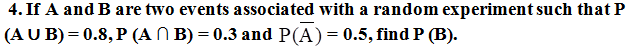Solution:

Given: A and B are two events.

P (A′) = 0.5, P (A ∩ B) = 0.3 and P (A ∪ B) = 0.8

Since, P (A′) = 1 – P (A)

P (A) = 1 – 0.5

= 0.5

Now we need to find P (B).

By definition of P (A or B) under axiomatic approach (also called addition theorem) we know that:

P (A ∪ B) = P (A) + P (B) – P (A ∩ B)

So, P (B) = P (A ∪ B) + P (A ∩ B) – P (A)

P (B) = 0.8 + 0.3 – 0.5

= 1.1 – 0.5

= 0.6

∴ P (B) is 0.6

5. Given two mutually exclusive events A and B such that P (A) = 1/2 and P (B) = 1/3, find P (A or B).

Solution:

Given: A and B are two mutually exclusive events.

P (A) = 1/2 and P (B) = 1/3

Now we need to find P (A ‘or’ B).

P (A or B) = P (A ∪ B)

So by definition of mutually exclusive events we know that:

P (A ∪ B) = P (A) + P (B)

= 1/2 + 1/3

= 5/6

∴ P (A ∪ B) is 5/6

6. There are three events A, B, C one of which must and only one can happen, the odds are 8 to 3 against A, 5 to 2 against B, fins the odds against C.

Solution:

As, out of 3 events A, B and C only one can happen at a time which means no event have anything common.

∴ We can say that A, B and C are mutually exclusive events.

So, by definition of mutually exclusive events we know that:

P (A ∪ B ∪ C) = P (A) + P (B) + P (C)

According to question one event must happen.

So, A or B or C is a sure event.

∴ P (A ∪ B ∪ C) = 1 … Equation (1)

We need to find odd against C:

Given:

Odd against A = 8/3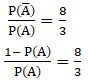8 P (A) = 3 – 3 P (A)

11 P (A) = 3

∴ P (A) = 3/11 … Equation (2)

Similarly, we are given with: Odd against B = 5/2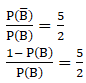5 P (B) = 2 – 2 P(B)

7 P (B) = 2

∴ P (B) =2/7 …Equation (3)

From equation 1, 2 and 3 we get:

P (C) = 1 – 3/11 – 2/7

= [77-21-22]/77

= 34/77

So, P (C′) = 1 – (34/77)

= 43/77

∴ Odd against C: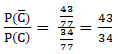7. One of the two events must happen. Given that the chance of one is two-third of the other, find the odds in favour of the other.

Solution:

Let A and B are two events.

As, out of 2 events A and B only one can happen at a time which means no event have anything common.

∴ We can say that A and B are mutually exclusive events.

So, by definition of mutually exclusive events we know that:

P (A ∪ B) = P (A) + P (B)

According to question one event must happen.

A or B is a sure event.

So, P (A ∪ B) = P (A) + P (B) = 1 … Equation (1)

Given: P (A) = (2/3) P (B)

We have to find the odds in favour of B.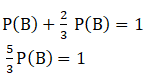P (B) = 3/5

So, P (B′) = 1 – 3/5

= 2/5

∴ Odd in favour of B: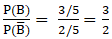8. A card is drawn at random from a well-shuffled deck of 52 cards. Find the probability of its being a spade or a king.

Solution:

Given: As a card is drawn from a deck of 52 cards.

Let ‘S’ denotes the event of card being a spade and ‘K’ denote the event of card being King.

As we know that a deck of 52 cards contains 4 suits (Heart, Diamond, Spade and Club) each having 13 cards. The deck has 4 king cards one from each suit.

We know that probability of an event E is given as-

By using the formula,

P (E) = favourable outcomes / total possible outcomes

= n (E) / n (S)

Where, n (E) = numbers of elements in event set E

And n (S) = numbers of elements in sample space.

Hence,

P (S) = n (spade) / total number of cards

= 13 / 52

= ¼

P (K) = 4/52

= 1/13

And P (S ⋂ K) = 1/52

We need to find the probability of card being spade or king, i.e.

P (Spade ‘or’ King) = P(S ∪ K)

So, by definition of P (A or B) under axiomatic approach (also called addition theorem) we know that:

P (A ∪ B) = P (A) + P (B) – P (A ∩ B)

So, P (S ∪ K) = P (S) + P (K) – P (S ∩ K)

= ¼ + 1/13 – 1/52

= 17/52 – 1/52

= 16/52

= 4/13

∴ P (S ∪ K) = 4/13

9. In a single throw of two dice, find the probability that neither a doublet nor a total of 9 will appear.

Solution:

In a single throw of 2 die, we have total 36(6 × 6) outcomes possible.

Say, n (S) = 36

Where, ‘S’ represents sample space

Let ‘A’ denotes the event of getting a double.

So, A = {(1,1), (2,2), (3,3), (4,4), (5,5), (6,6)}

P (A) = n (A) / n (S)

= 6/36

= 1/6

And ‘B’ denotes the event of getting a total of 9

So, B = {(3,6), (6,3), (4,5), (5,4)}

P (B) = n (B) / n (S)

= 4/36

= 1/9

We need to find probability of neither the event of getting neither a doublet nor a total of 9.

P (A′ ∩ B′) =?

As, P (A′ ∩ B′) = P (A ∪ B)′ {using De Morgan’s theorem}

P (A′ ∩ B′) = 1 – P (A ∪ B)

By using the definition of P (E or F) under axiomatic approach (also called addition theorem) we know that:

P (E ∪ F) = P (E) + P (F) – P (E ∩ F)

∴ P (A ∪ B) = 1/6 + 1/9 + 0

= 5/18 {Since, P (A ∩ B) = 0 since nothing is common in set A and B.

So, n (A ∩ B) = 0}

Hence,

P (A′ ∩ B′) = 1 – (5/18)

= 13/18

10. A natural number is chosen at random from amongst first 500. What is the probability that the number so chosen is divisible by 3 or 5?

Solution:

Given: Sample space is the set of first 500 natural numbers.

n (S) = 500

Let ‘A’ be the event of choosing the number such that it is divisible by 3

n (A) = [500/3]

= [166.67]

= 166 {where [.] represents Greatest integer function}

P (A) = n (A) / n (S)

= 166/500

= 83/250

Let ‘B’ be the event of choosing the number such that it is divisible by 5

n (B) = [500/5]

= 

= 100 {where [.] represents Greatest integer function}

P (B) = n (B) / n (S)

= 100/500

= 1/5

Now, we need to find the P (such that number chosen is divisible by 3 or 5)

P (A or B) = P (A ∪ B)

By using the definition of P (E or F) under axiomatic approach (also called addition theorem) we know that:

P (E ∪ F) = P (E) + P (F) – P (E ∩ F)

∴ P (A ∪ B) = P (A) + P (B) – P (A ∩ B)

[Since, we don’t have value of P(A ∩ B) which represents event of choosing a number such that it is divisible by both 3 and 5 or we can say that it is divisible by 15.]

n(A ∩ B) = [500/15]

= [33.34]

= 33

P (A ∩ B) = n(A ∩ B) / n (S)

= 33/500

∴ P (A ∪ B) = P (A) + P (B) – P (A ∩ B)

= 83/250 + 1/5 – 33/500

= [166 + 100 – 33]/500

= 233/500

11. A die is thrown twice. What is the probability that at least one of the two throws come up with the number 3?

Solution:

If a dice is thrown twice, it has a total of (6 × 6) = 36 possible outcomes.

If S represents the sample space then,

n (S) = 36

Let ‘A’ represent events the event such that 3 comes in the first throw.

A = {(1,3), (2,3), (3,3), (4,3), (5,3), (6,3)}

P (A) = n (A) / n (S)

= 6 /36

= 1/6

Let ‘B’ represent events the event such that 3 comes in the second throw.

B = {(3,1), (3,2), (3,3), (3,4), (3,5), (3,6)}

P (B) = n (B) / n (S)

= 6 /36

= 1/6

It is clear that (3,3) is common in both events so,

P (A ∩ B) = n (A ∩ B) / n (S)

= 1/ 36

Now we need to find the probability of event such that at least one of the 2 throws give 3 i.e. P (A or B) = P (A ∪ B)

By using the definition of P (E or F) under axiomatic approach (also called addition theorem) we know that:

P (E ∪ F) = P (E) + P (F) – P (E ∩ F)

So, P (A ∪ B) = P (A) + P (B) – P (A ∩ B)

= 1/6 + 1/6 – 1/36

= 1/3 – 1/36

= 11/36

∴ P (at least one of the two throws comes to be 3) is 11/36

12. A card is drawn from a deck of 52 cards. Find the probability of getting an ace or a spade card.

Solution:

Given: As a card is drawn from a deck of 52 cards.

Let ‘S’ denotes the event of card being a spade and ‘K’ denote the event of card being ace.

As we know that a deck of 52 cards contains 4 suits (Heart, Diamond, Spade and Club) each having 13 cards. The deck has 4 ace cards one from each suit.

We know that probability of an event E is given as-

By using the formula,

P (E) = favourable outcomes / total possible outcomes

= n (E) / n (S)

Where, n (E) = numbers of elements in event set E

And n (S) = numbers of elements in sample space.

Hence,

P (S) = n (spade) / total number of cards

= 13 / 52

= ¼

P (K) = 4/52

= 1/13

And P (S ⋂ K) = 1/52

We need to find the probability of card being spade or ace, i.e.

P (Spade ‘or’ ace) = P(S ∪ K)

So, by definition of P (A or B) under axiomatic approach (also called addition theorem) we know that:

P (A ∪ B) = P (A) + P (B) – P (A ∩ B)

So, P (S ∪ K) = P (S) + P (K) – P (S ∩ K)

= ¼ + 1/13 – 1/52

= 17/52 – 1/52

= 16/52

= 4/13

∴ P (S ∪ K) = 4/13

13. The probability that a student will pass the final examination in both English and Hindi is 0.5 and the probability of passing neither is 0.1. If the probability of passing the English examination is 0.75. What is the probability of passing the Hindi examination?

Solution:

Let ‘E’ denotes the event that student passes in English examination.

And ‘H’ be the event that student passes in Hindi exam.

It is given that,

P (E) = 0.75

P (passing both) = P (E ∩ H) = 0.5

P (passing neither) = P (E′ ∩ H′) = 0.1

P (H) =?

As, we know that P (A′ ∩ B′) = P (A ∪ B) ′ {using De Morgan’s law}

P (E′ ∩ H′) = P (E ∪ H)′

0.1 = 1 – P (E ∪ H)

P (E ∪ H) = 1 – 0.1

= 0.9

By using the definition of P (A or B) under axiomatic approach (also called addition theorem) we know that:

P (A ∪ B) = P (A) + P (B) – P (A ∩ B)

∴ P (E ∪ H) = P (E) + P (H) – P (E ∩ H)

0.9 = 0.75 + P (H) – 0.5

1.4 – 0.75 = P (H)

∴ P (H) = 0.65

14. One number is chosen from numbers 1 to 100. Find the probability that it is divisible by 4 or 6?

Solution:

Given: Sample space is the set of first 100 natural numbers.

n (S) = 100

Let ‘A’ be the event of choosing the number such that it is divisible by 4

n (A) = [100/4]

= 

= 25 {where [.] represents Greatest integer function}

P (A) = n (A) / n (S)

= 25/100

= ¼

Let ‘B’ be the event of choosing the number such that it is divisible by 6

n (B) = [100/6]

= [16.67]

= 16 {where [.] represents Greatest integer function}

P (B) = n (B) / n (S)

= 16/100

= 4 /25

Now, we need to find the P (such that number chosen is divisible by 4 or 6)

P (A or B) = P (A ∪ B)

By using the definition of P (E or F) under axiomatic approach (also called addition theorem) we know that:

P (E ∪ F) = P (E) + P (F) – P (E ∩ F)

∴ P (A ∪ B) = P (A) + P (B) – P (A ∩ B)

[Since, we don’t have the value of P (A ∩ B) which represents event of choosing a number such that it is divisible by both 4 and 6 or we can say that it is divisible by 12.]

n (A ∩ B) = [100/12]

= [8.33]

= 8

P (A ∩ B) = n (A ∩ B) / n (S)

= 8/100

= 2/25

∴ P (A ∪ B) = P (A) + P (B) – P (A ∩ B)

P (A ∪ B) = ¼ + 4/25 – 2/25

= ¼ + 2/25

= 33/100